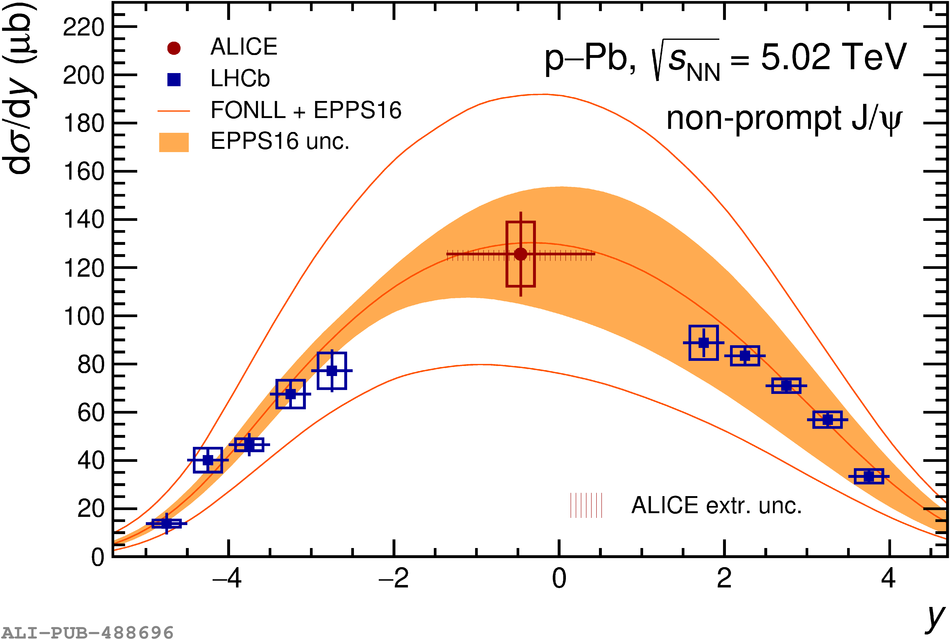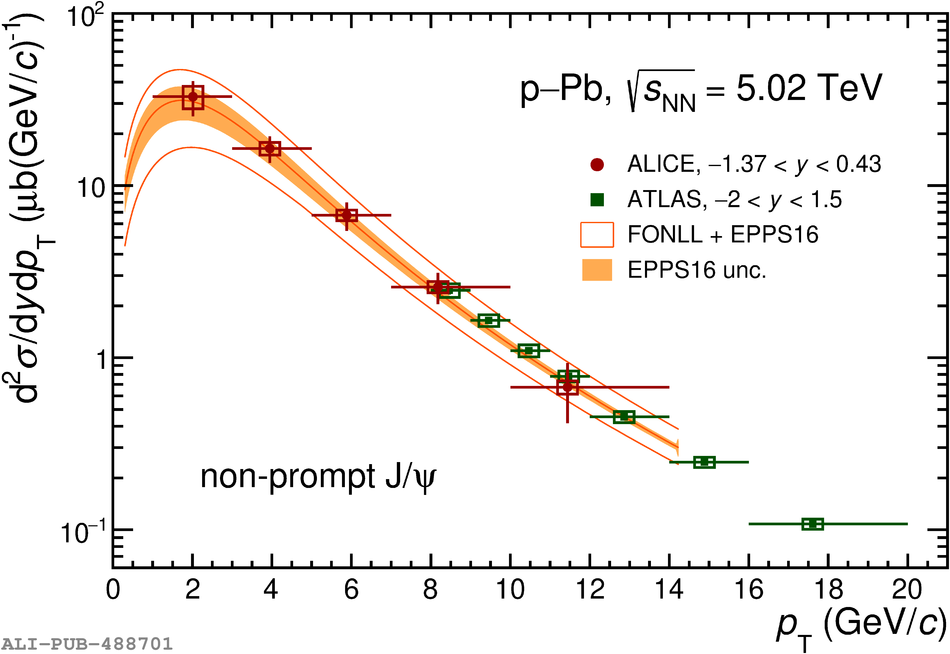# Figure 5

 $\textup{d}\sigma_{\rm \JJpsi\ from\ h_{\rm b}}/\textup{d}y$ as a function of rapidity (left panel) as obtained at midrapidity in this work and by the LHCb collaboration in the forward and backward rapidity regions , and $\textup{d}^2\sigma_{\rm \JJpsi\ from\ h_{\rm b}}/\textup{d}y\textup{d}p_{\rm T}$ as a function of \pt (right panel) compared with ATLAS measurements  (shown up to $\pt = 20$ GeV/$c$). The statistical and systematic uncertainties are shown as vertical error bars and hollow boxes, respectively. For the measurements as a function of \pt, data symbols are placed at the mean value of the \pt distribution within each bin The results are compared to FONLL computations  with EPPS16  nPDFs, highlighting the total theoretical uncertainty (empty band) and the contribution from EPPS16 (coloured band). In the right panel, model computations are obtained in the same rapidity range of the ALICE results.